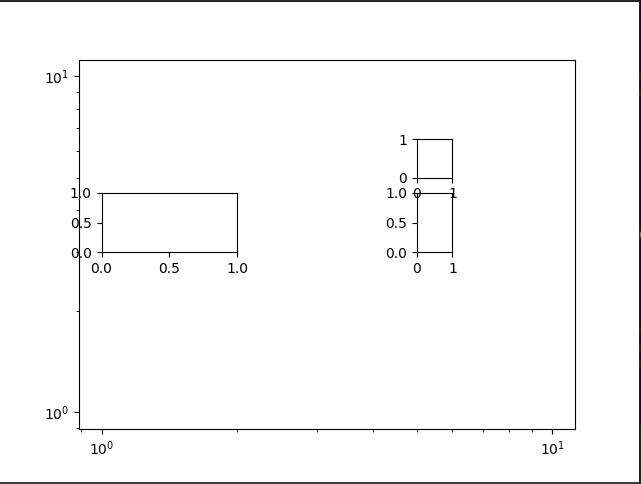# matplotlib.axes.Axes.inset_axes

How to make the inset_axes in the correct position if the x- and y-axis scales are not the same.

not sure what is mean by “correct position”. Note inset axes can take a transform kwarg and even a blended transform.

``````import numpy as np
import matplotlib.pyplot as plt
import matplotlib.image as mpimg

x = np.arange(0, 10, 0.005)
y = np.exp(-x/2.) * np.sin(2*np.pi*x)

fig = plt.figure()
ax.plot(x, y)
ax.set_xlim(0, 10)
ax.set_ylim(-1, 1)

axin = ax.inset_axes([4, 0, 1, 1], transform=ax.transData)
axin.imshow(im)
axin.set_xticks([])
axin.set_yticks([])

plt.show()
``````

The inserted picture is not in the position with coordinate (4, 0)，What should I do?

In display space, your selected region is very tall compared to its width. Calling `imshow` sets the Axes to a square aspect ratio. So the Axes did start at (4, 0), but the image is only displayed in a square region that fits in its bounds, which is halfway between 0 and 1 because it’s centered.

Thank you very much, I got it.

I have another question. If the x-axis and y-axis are set like this(`ax.set_xscale('log'), ax.set_yscale('log')`), can the length and width be fixed? When changing the position, the size of the picture is also changing, so I want to fix the size of the picture and the coordinate position is correct

The length and width of what?

I do not understand the question.

``````import numpy as np
import matplotlib.pyplot as plt

fig = plt.figure()

ax.set_xscale('log')
ax.set_yscale('log')

axin1 = ax.inset_axes([1, 3, 1, 1.5], transform=ax.transData)
axin2 = ax.inset_axes([5, 3, 1, 1.5], transform=ax.transData)
axin3 = ax.inset_axes([5, 5, 1, 1.5], transform=ax.transData)

plt.show()
``````I’m sorry I didn’t make it clear, Due to the logarithm, the width and height of each inset_axes will be different even if they take the same value. I wonder if you can fix a width and height to keep the size of inset_axes unchanged

If you change to transaxes, the coordinates are difficult to be in the specified position. I think about it these days. I can calculate the logarithm directly without setting it to `ax.set_xscale('log')`